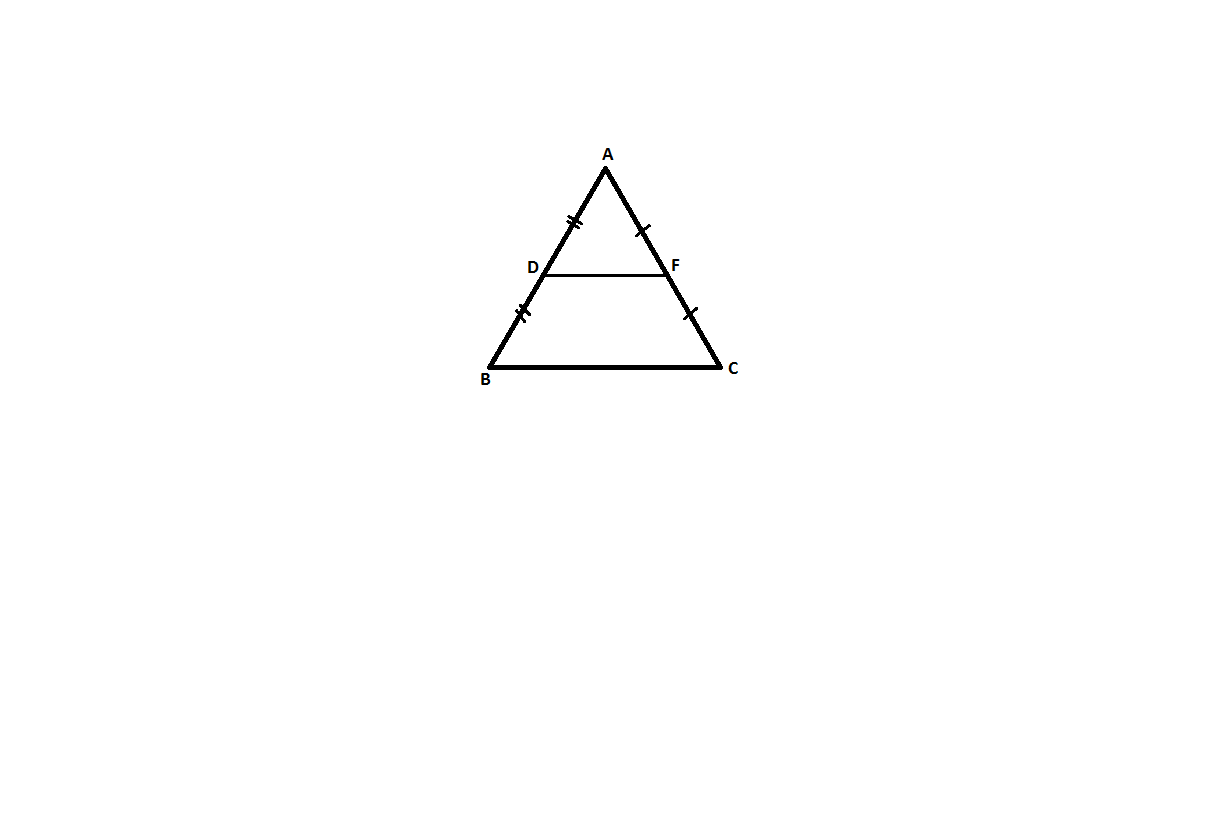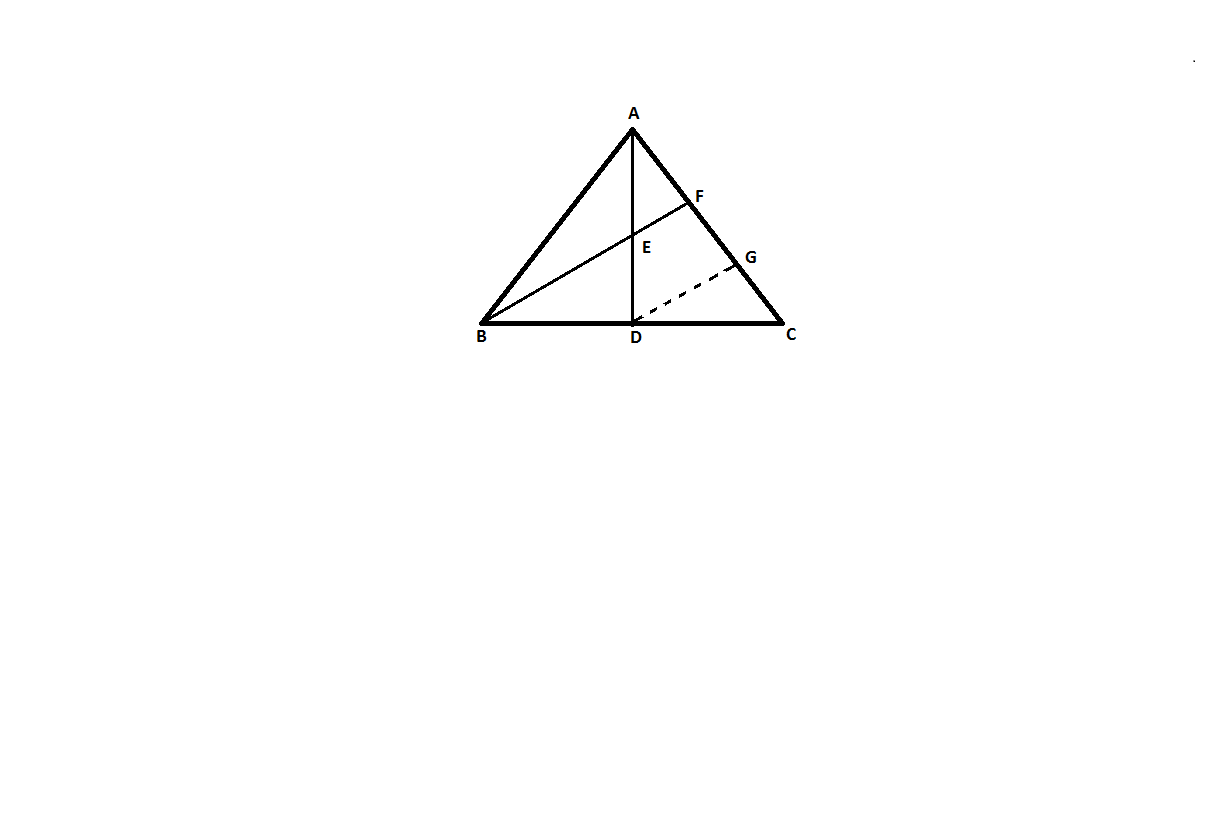QuestionAnswers

# In a triangle ABC, AD is a median and E is the midpoint of median AD. A line through B and E meets AC at point F. Prove that $AC = 3AF$Hint:For this question mid-point theorem is to be used.
Mid-point theorem: - It says a line segment joining the mid-points of two sides of a triangle is parallel to the third side and equal to half the length of the third side.
Example: -In triangle ABC, D is midpoint of AB and E is midpoint of AC.
$\therefore DF//BC\\ \therefore DF = \dfrac{1}{2}BC$
So we can say that it bisects the line into two equal parts.

Construction: - Draw DG parallel to BF, which meets FC at G.Proof: - In triangle ABC, AD is median and E is the midpoint of AD.
In and E is the midpoint of AD. Hence, F is midpoint of AG (midpoint theorem)
Or, $AF = FG$ ................equation(1)
In and D is the midpoint of BC.
$\therefore$ G is midpoint of CF (midpoint theorem)
Or, $FG = GC$ .................equation(2)
From equation (1) and (2)
$AF = FG = GC$
Since: - $AC = AF + FG + GC$
$AC = 3AF$
Hence proved.

Note: Carefully observe the hint which is given in the question and then proceed for the question. Here $DG\parallel BF$ gives a hint that the mid- point theorem is to be used. Similar questions can be framed with the use of a converse mid-point theorem.
Converse mid-point theorem: - It states that when a line is drawn through the midpoint of a side of a triangle which is parallel to the second side then it will bisect the third side.
View Notes
Where There is a Will There is a Way EssayThe Difference Between an Animal that is A Regulator and One that is A ConformerLaughter is a Best Medicine EssayFunctions of vitamin A, B, C, D, ERelation Between Mean Median and ModeThe Making of a ScientistWhat is the full form of phd?Changing the Period of a PendulumWhat is the Difference Between Trade and Commerce?CBSE Class 7 Maths Chapter 6 - Triangle and Its Properties FormulasImportant Questions for CBSE Class 6 English A Pact with The Sun Chapter 10 - A Strange Wrestling MatchImportant Questions for CBSE Class 6 English A Pact with The Sun Chapter 8 - A Pact with the SunImportant Questions for CBSE Class 6 English A Pact with The Sun Chapter 1 - A Tale of Two BirdsImportant Questions for CBSE Class 7 Social Science - Social and Political Life Chapter 8 - A Shirt In The MarketImportant Questions for CBSE Class 6 English A Pact with The Sun Chapter 6 - The Monkey and the CrocodileImportant Questions for CBSE Class 6 English A Pact with The SunImportant Questions for CBSE Class 11 Physics Chapter 4 - Motion in a PlaneImportant Questions for CBSE Class 7 English An Alien Hand Chapter 9 - A Tiger In The HouseImportant Questions for CBSE Class 6 English A Pact with The Sun Chapter 2 - The Friendly MongooseImportant Questions for CBSE Class 6 English A Pact with The Sun Chapter 3 - The Shepherd's TreasureCBSE Class 10 Hindi A Question Paper 2020Hindi A Class 10 CBSE Question Paper 2009Hindi A Class 10 CBSE Question Paper 2015Hindi A Class 10 CBSE Question Paper 2016Hindi A Class 10 CBSE Question Paper 2012Hindi A Class 10 CBSE Question Paper 2010Hindi A Class 10 CBSE Question Paper 2007Hindi A Class 10 CBSE Question Paper 2013Hindi A Class 10 CBSE Question Paper 2008Hindi A Class 10 CBSE Question Paper 2014RS Aggarwal Class 9 Solutions Chapter-9 Congruence of Triangles and Inequalities in a TriangleNCERT Solutions for Class 9 English Moments Chapter 8 - A House is Not a HomeRS Aggarwal Class 11 Solutions Chapter-16 Conditional Identities Involving the Angles of a TriangleNCERT Solutions Class 11 English Woven Words Essay Chapter 5 What is a Good BookRS Aggarwal Class 8 Mathematics Solutions for Chapter-18 Area of a Trapezium and a PolygonNCERT Solutions for Class 7 Maths Chapter 6 The Triangle and Its Properties In HindiNCERT Solutions for Class 7 Maths Chapter 6 The Triangle and Its PropertiesNCERT Solutions for Class 12 English Kaliedoscope Poetry Chapter 4 Kubla Khan or a Vision in a Dream a FragmentRS Aggarwal Class 10 Solutions - Mean, Median, Mode of Grouped Data, Cumulative Frequency Graph and OgiveNCERT Solutions for Class 10 Social Science India and the Contemporary World - II Chapter 4 - The Making of a Global World